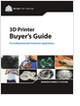Formula HomeBuckling of ColumnsIntroductionCritical LoadElastic BucklingInelastic BucklingColumn RelatedEccentric LoadsInitially CurvedBeam-ColumnsCalculatorsCritical LoadStruc. Steel ColumnsRelated SubjectsMaterials MechanicsBeamsResourcesBibliography
Essentials of Manufacturing

Information, coverage of important developments and expert commentary in manufacturing.

3D Scanners

A white paper to assist in the evaluation of 3D scanning hardware solutions.

Selecting the Right 3D Printer

Discover how to choose the right 3D printer for your needs and the key performance attributes to consider.

Mechanical Engineers Outlook

Guide for those interested in becoming a mechanical engineer. Includes qualifications, pay, and job duties.

more free publicationsEccentric Axial LoadIn the case of an ideal column under an axial load, the column remains straight until the critical load is reached. However, the load is not always applied at the centroid of the cross section, as is assumed in Euler buckling theory. This section analyzes a simply-supported column under an eccentric axial load. Consider a column of length L subject to an axial force F. On one end of the column, the force F is applied a distance e from the central column axis, as shown in the schematic below.Balance the moments on the free-body diagram on the right requires that,The governing equation for the column's transverse displacement w can then be written as,where M was eliminated using Euler-Bernoulli beam theory. The above equation contains a non-homogeneous term -Fe/EI and its general solution is,where. The coefficients A and B depend on the boundary conditions. For a simply supported column the boundary conditions are,The solution for the column's displacement is therefore,where.
Secant FormulaIn practice, engineers are usually interested in the maximum stress rather than the displacement curve alone. The secant formula discussed in this section derives the maximum stress from the displacement formula obtained in the previous section.

The normal stress in the column results from both the direct axial load F and the bending moment M resulting from the eccentricity e of the force application,where A is the cross-section area, and I is the moment of inertia of the cross section.

The maximum stress is located at the extreme fiber on the concave side (y = c) of the middle point (x = L/2) of the column,where,(obtained by applying basic trigonometric relations to the displacement formula in the previous section). The parameter c is the distance from the centroidal axis to the extreme fiber on the concave side of the column.

Expanding the formula for the maximum stress, we have,The radius of gyration r is defined as. Working r into the above stress equation results in the secant formula for maximum stress,The secant formula indicates that in addition to the axial load F and cross-section area A, the maximum stress also depends on the eccentricity ratio ec/r2 and the slenderness ratio L/r.

 Note: 1 The secant formula can be used to compute the allowable normal stress for a given design,where sallow is the maximum allowable stress (e.g. yield stress). 2 If the eccentricity e is zero, the secant formula no longer applies. In this case Euler's formula must be used for slender beams.GlossaryEssentials of Manufacturing

Information, coverage of important developments and expert commentary in manufacturing.3D Scanners

A white paper to assist in the evaluation of 3D scanning hardware solutions.Selecting the Right 3D Printer

Discover how to choose the right 3D printer for your needs and the key performance attributes to consider.Mechanical Engineers Outlook

Guide for those interested in becoming a mechanical engineer. Includes qualifications, pay, and job duties.